# Exploring Slope and Y-Intercept • Teacher Dashboard

Excite Bike Equations: Exploring Slope and Y-Intercept
cxfx6m
SnapshotsSummaryTeacherStudent
Anonymize
Pacing
Pause
0 students
Time EnteredExcitebike is a video game where players race a motorcycle and jump over ramps. What do you notice about the different ramps?
1Introduction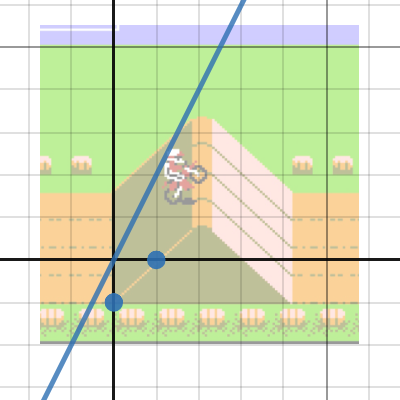2First Jump. Can you move the sliders to make the line go through the two points?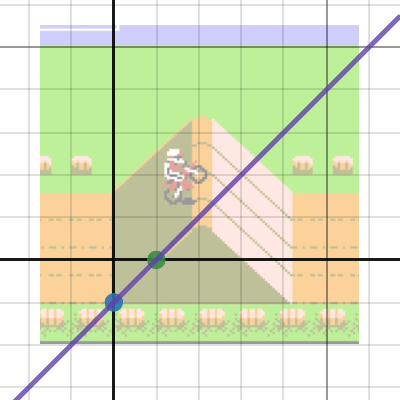What equation did you get for this line?
3First Jump. What equation did you get?4First Jump. Can you move the sliders to make the line go through the two points?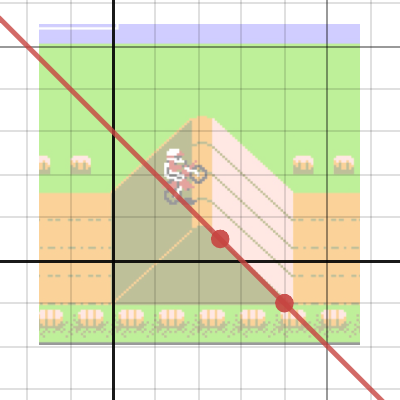What equation did you get for this line? How is different from the first one?
5First Jump.How does this jump compare to the first one? What do you think that means for the slope of the ramp?
6Second Jump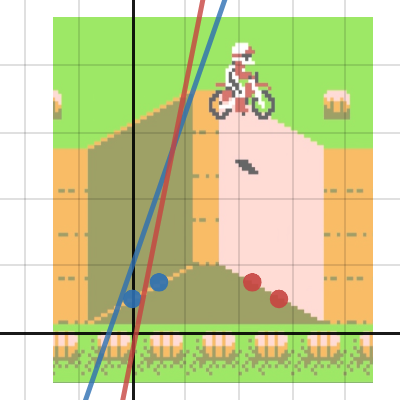72nd Jump. Can you find the equations of the blue and red lines through the matching points?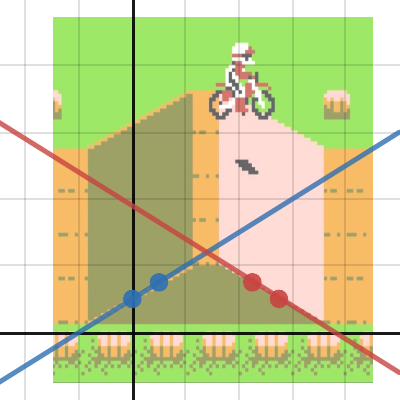What equations did you get for the blue and red lines?
82nd Jump. Can you find the equations of the blue and red lines through the matching points?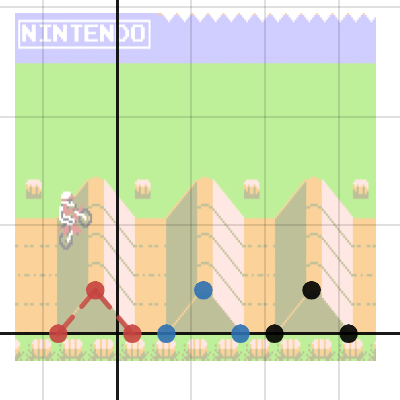9Find the equations that will make the other two ramps. Use the given line segments as a guide.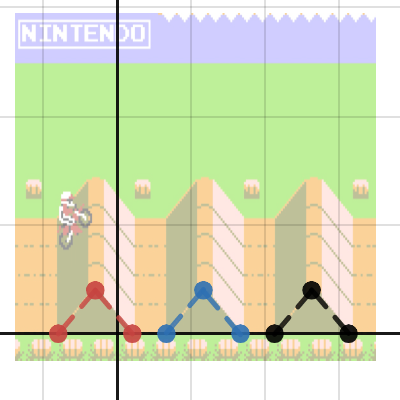What did you notice about the slopes of all the up ramps in the last slide? The slopes of the down ramps?
10Find the equations that will make the other two ramps. Use the given line segments as a guide.